﻿ 基于平滑先验法的两种熵研究 Two Entropy Studies Based on Smooth Prior Approach

Biophysics
Vol. 07  No. 01 ( 2019 ), Article ID: 28723 , 9 pages
10.12677/BIPHY.2019.71001

Two Entropy Studies Based on Smooth Prior Approach

Yuanyuan Guo*, Jin Li#, Dezhao Jiao

School of Physics and Information Technology, Shaanxi Normal University, Xi’an ShaanxiReceived: Jan. 11th, 2019; accepted: Jan. 23rd, 2019; published: Jan. 30th, 2019ABSTRACT

The heart rate variability (HRV) signals collected from the ECG system are affected with noise and various trends inevitably. Eliminating the interference of these trends contributes to the accuracy of complex dynamic analysis of these nonlinear systems. In this paper, we use the smoothness prior method to eliminate the different trends superimposed in the HRV signals of healthy people during the day and night, and use the approximate entropy and the basic scale entropy to calculate the complexity. Before and after removing the trending interference, we contrast and analyze the stability changes of approximate entropy and base-scale entropy. The results showed that after detrending processing, the smooth prior method can effectively remove various trend interference from the recordings, and can greatly improve the stability of approximate entropy. The experimental results also showed that the base-scale entropy indicates strong stability regardless of whether or not to use detrending processing. After detrending, the two entropy measures can distinguish the HRV signals of healthy people during the day and night. It fully reflects that the smoothness prior method can improve the stability and recognition of the entropy measure, and provide an important theoretical basis for clinical physiology and pathological diagnosis.

Keywords:Smoothness Prior Method, Heart Rate Variability, Base-Scale Entropy, Approximate Entropy, Stability1. 引言

2. 理论基础

2.1. 平滑先验法及其频率响应

2.1.1. 平滑先验法原理

$z={z}_{s}+{z}_{t}$ (1)

${z}_{t}=H\theta +v$ (2)

${\theta }_{\lambda }=\mathrm{arg}\underset{\theta }{\mathrm{min}}\left\{{‖H\theta -z‖}^{2}+{\lambda }^{2}{‖{D}_{d}\left(H\theta \right)‖}^{2}\right\}$ (3)

${R}_{2}={\left[{R}_{3}-{R}_{2}-\left({R}_{2}-{R}_{1}\right)\text{,}{R}_{4}-{R}_{3}-\left({R}_{3}-{R}_{2}\right),\cdots ,{R}_{N}-{R}_{N-1}-\left({R}_{N-1}-{R}_{N-2}\right)\right]}^{T}$ (4)

${D}_{d}=\left[\begin{array}{ccc}d{\left({R}_{d}\right)}_{1}/d{R}_{1}& \cdots & d{\left({R}_{d}\right)}_{1}/d{R}_{N}\\ ⋮& & ⋮\\ d{\left({R}_{d}\right)}_{N-d}/d{R}_{1}& \cdots & d{\left({R}_{d}\right)}_{N-d}/d{R}_{N}\end{array}\right]$ (5)

${D}_{2}=\left[\begin{array}{cccccc}1& -2& 1& 0& \cdots & 0\\ 0& 1& -2& 1& \cdots & ⋮\\ ⋮& ⋮& ⋮& ⋮& & 0\\ 0& \cdots & 0& 1& -2& 1\end{array}\right]$ (6)

$\stackrel{^}{{z}_{s}}=z-H{\stackrel{^}{\theta }}_{\lambda }=\left[I-{\left(I+{\lambda }^{2}{D}_{2}^{T}{D}_{2}\right)}^{-1}\right]z=Lz$ (7)

2.1.2. 频率响应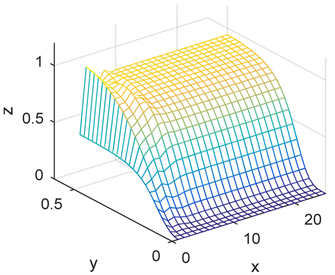Figure 1. Frequency response of L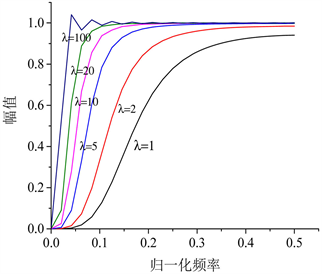Figure 2. Frequency response at different λTable 1. Regularization parameter and the corresponding cut-off frequency

2.2. 近似熵

2.3. 基本尺度熵

3. HRV信号的去趋势项及仿真分析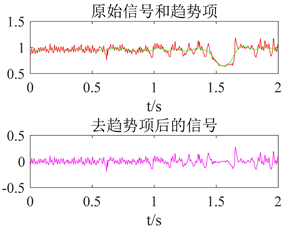(a) 健康人白天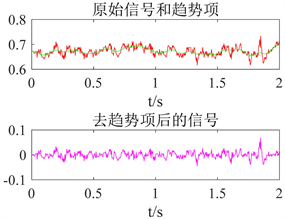(b) 健康人夜间

Figure 3. The waveform before and after detrending using smoothness priors approach

4. 基于SPA算法的两种熵分析Figure 4. Base-scale entropy of two sets of samples before detrending processing (a) and after detrending processing (b)Table 2. Base-scale entropy statistical analysis of HRV signals (mean ± standard)Table 3. Base-scale entropy statistical analysis of HRV signals after detrending processing (mean ± standard)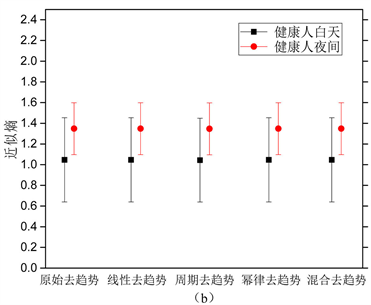Figure 5. Approximate entropy of two sets of samples before detrending processing (a) and after detrending processing (b)Table 4. Approximate entropy statistical analysis of HRV signalsTable 5. Approximate entropy statistical analysis of HRV signals after detrending processing

5. 结论

Two Entropy Studies Based on Smooth Prior Approach[J]. 生物物理学, 2019, 07(01): 1-9. https://doi.org/10.12677/BIPHY.2019.71001

1. 1. Acharya, U.R., Joseph, K.P., Kannathal, N., Lim, C.M. and Suri, J.S. (2006) Heart Rate Variability: A Review. Medical & Biological Engineering & Computing, 44, 1031-1051. https://doi.org/10.1007/s11517-006-0119-0

2. 2. Mendia-Iztueta, I., Monahan, K., Kyrolainen, H., et al. (2016) Assessment of Heart Rate Variability Thresholds from Incremental Treadmill Tests in Five Cross-Country Skiing Techniques. PloS One, 11, e0145875. https://doi.org/10.1371/journal.pone.0145875

3. 3. 宁新宝. 生物医学信号时间属性及其分析研究的进展[J]. 数据采集与处理, 2013, 28(5): 529-538.

4. 4. 张璇, 李锦, 徐文敏. 昼夜节律颠倒影响心率变异性信号的非线性特性研究[J]. 陕西师范大学学报(自科版), 2016, 44(2): 48-53.

5. 5. Matsoukas, C., Islam, S. and Iturbe, I.R. (2000) Detrended Fluctuation Analysis of Rainfall and Streamflow Tine Series. Journal of Geophysical Research, 105, 29165-29172. https://doi.org/10.1029/2000JD900419

6. 6. Gu H, and Song B F. (2009) Study on Effectiveness Evaluation of Weapon Systems Based on Grey Relational Analysis and TOPSIS. Journal of Systems Engineering and Electronics, 20, 106-111.

7. 7. Huang, N.E., Shen, Z., Long, S.R., et al. (1998) The Empirical Mode Decomposition and the Hilbert Spectrum for Nonlinear and Non-Stationary Time Series Analysis. Proceedings of the Royal Society of London Series A, 454, 903-995. https://doi.org/10.1098/rspa.1998.0193

8. 8. Tarvainen, M.P., Ranta-Aho, P.O. and Karjalainen, P.A. (2002) An Advanced Detrending Method with Application to HRV Analysis. IEEE Transactions on Biomedical Engineering, 49, 172-175. https://doi.org/10.1109/10.979357

9. 9. 李锦, 宁新宝. 短时心率变异性信号的基本尺度熵分析[J]. 科学通报, 2005, 50(14): 1438-1441.

10. 10. Karjalainen, P.A. (1997) Regulatization and Bayesian Methods for Evoked Potential Estimation. Kuopio University, Kuopio, 50-52.

11. 11. 杨红超. 基于移动平台的心电信号实时监控系统[D]: [硕士学位论文]. 上海: 东华大学, 2013.

12. 12. Pincuse, S.M. (1991) Approximate Entropy as a Measure of System Complexity. Proceedings of the National Academy of Sciences of the United States of America, 88, 2297-2301. https://doi.org/10.1073/pnas.88.6.2297

13. 13. Pincuse, S.M. and Goldberger, A.L. (1994) Physiological Time-Series Analysis: What Does Regularity Quantify? American Journal of Physiology-Heart and Circulatory Physiology, 266, 1643-1656.

14. 14. Li, J. and Ning, X.B. (2005) The Base-Scale Entropy Analysis of Short-Time Heart Rate Variability Signal. Chinese Science Bulletin, 50, 1269-1273. https://doi.org/10.1360/982005-94

15. 15. 李锦, 刘大钊. 昼夜节律下心率变异性信号的熵信息和谱特征[J]. 物理学报, 2012, 61(20): 547-552.

16. 16. Wessel, N., Ziehmann, C., Kurths, J., Meyerfeldt, U., Schirdewan, A. and Voss, A. (2000) Short-Term Forecasting of Life-Threatening Cardiac Arrhythmias Based on Symbolic Dynamics and Fi-nite-Time Growth Rates. Physical Review E, 61, 733.

17. 17. Scheer, F.A., van Doornen, L.J. and Buijs, R.M. (1999) Light and Diurnal Cycle Affect Human Heart Rate: Possible Role for the Circadian Pacemaker. Journal of Biological Rhythms, 14, 202-212. https://doi.org/10.1177/074873099129000614

18. 18. Guo, Y.F. and Stein, P.K. (2003) Circadian Rhythm in the Cardiovascular System: Chronocardiology. American Heart Journal, 145, 779-786. https://doi.org/10.1016/S0002-8703(02)94797-6

19. 19. Jin, L. and Jun, W. (2013) Entropy Information of Heart Rate Variability and Its Power Spectrum during Day and Night. Europhysics, 103, Article ID: 28002.

20. 20. 李镒冲, 李晓松. 两种测量方法定量测量结果的一致性评价[J]. 现代预防医学, 2007, 34(17): 3263-3266.

21. NOTES

*第一作者。

#通讯作者。